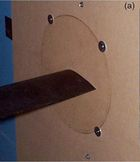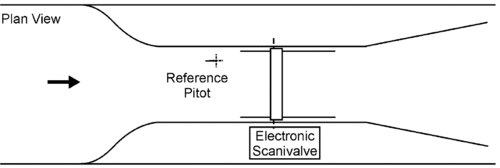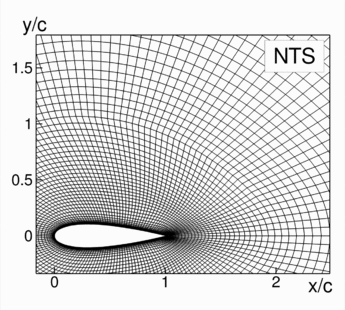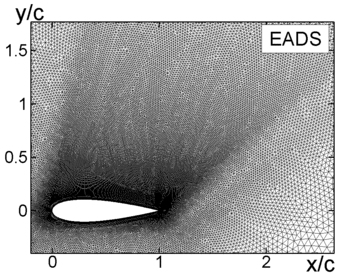# Test Case Study

## Brief Description of the Test Case

The following presents a precise description of the primary test case, the NACA0021 airfoil at 60° angle of attack.

A visual impression of the geometry and flow has been shown in Figure 1. The experiments were carried out in the wind tunnel of Monash University (see Figure 2). The width of the experimental section is 7.2 airfoil chord lengths, c, and its height is 16c.

 Figure 2: NACA0021 airfoil in wind tunnel (left) and a plan view of wind tunnel (right) [‌27]The airfoil geometry normalized with the chord length, c, is defined by:

${y=\pm {\frac {0.21}{0.2}}\left(0.2969\ {\sqrt {x}}-0.126\ x-0.3516\ x^{2}+0.2846\ x^{3}-0.1015\ x^{4}\right)}$Experimental flow parameters, needed to set up appropriate numerical simulations, are presented in Table 2.

Table 2: Flow parameters
Parameter Notation Value
Reynolds number ${Re=U_{\infty }c/\nu }$2.7×105
Chord length $\left.c\right.$0.125 m
Angle of attack $\left.\alpha \right.$60°
Free stream Mach number $\left.M\right.$0.1
Free stream streamwise turbulence intensity $\left.l_{u}\right.$0.6%

The flow parameters measured in the experiments are as follows:

• Time-averaged pressure coefficient distribution over the airfoil surface, ${{\overline {C_{p}}}(x)={\frac {p-p_{\infty }}{{\frac {1}{2}}\rho U_{\infty }^{2}c}}}$, where $\left.p_{\infty }\right.$is the reference pressure from the undisturbed far-field flow and $\left.\rho \right.$is the fluid density.

• Time-averaged sectional drag and lift coefficients, $\left.{\overline {C_{d}}},\ {\overline {C_{l}}}\right.$integrated from pressure at individual spanwise locations near the spanwise mid-point: ${C_{d}={\frac {d}{{\frac {1}{2}}\rho U_{\infty }^{2}c}},\ C_{l}={\frac {l}{{\frac {1}{2}}\rho U_{\infty }^{2}c}}}$, where $\left.d\right.$and $\left.l\right.$are the sectional pressure drag and lift forces, respectively.

• Time histories of the sectional lift and drag coefficients (32,000 points total over the time interval T≈9000 (c/U0)).

The experimental data of Swalwell [‌28] for NACA0021 at 60° angle of attack, Re = 2.7×105, are available here:

• Avg_surface_CP.dat
Mean surface pressure coefficient distribution. Column 1: tapping position along chordwise (x) axis. Following columns: mean ${\overline {C_{P}}}$at 45°, 60° and 90° angle of attack, taken from two different pressure tapping rows (denoted B1 and B2).

• CT_nondim_NACA0021_60deg.dat
Time traces of each force coefficient (L = lift, D = drag, N = chord normal, T = chord tangential). Column one: dimensionless time (chord / onflow velocity), column two: force coefficient.

• PSD_exp_Cl_-l909_-s10_-v.dat
Power spectral density of force coefficients. These employ Welch's averaged periodogram method with windows of length 909 samples and a shift between windows of 10 samples. The Hanning window function was used.

## Test Case Experiments

A detailed description of the test facility and measurement techniques used in the experiments is given in [‌27, 28]. So here we present only concise information about these aspects of the test case.

As already mentioned, the width of the experimental section is 7.2 airfoil chord lengths c and its height is 16c.The two-dimensionality of the flow over the NACA0021 model was improved by the use of the endplates (see Figure 2). It was found that the free-stream flow has a turbulence intensity of 0.6% and variations of the velocity over the central 0.3m×0.3m area of the test section are less than 3%. During the runs the dynamic pressure was determined by a Pitot upstream of and above the model. This allowed the coefficient of pressure to be determined for each sample. Although the flow decelerates over the distance from the Pitot to the section containing the model, an error in velocity caused by this was less than 6% and was not corrected for.

The aspect ratio of the model was equal to 7.2, which ensured a low value of blockage (6.25% at α = 90°) and is sufficient to minimize possible effects of the finite span on the unsteady flow characteristics [‌27].

The time-averaged pressure coefficient distribution over the airfoil surface was measured with the use of multiple pressure taps arranged in five rows along the model span. In the streamwise direction the taps were concentrated towards the leading edge, which allowed a better resolution of the high pressure gradients in this area. The model was aligned to zero angle of attack by equalizing the pressure on its top and bottom surface. During the runs each tap was sampled at 1000 Hz for 35 seconds and measured pressure signals were corrected for the amplitude and phase response of the tubing. The corrected pressure measurements were fitted with a spline function across the surface for integration of the forces.

The lift and drag were computed from the measured pressures for each time step and then analyzed for frequency content, which resulted in the sectional PSD of the forces. Thus both mean and time-dependent forces are available.

## CFD Methods

During the DESider project the considered test case was computed by 8 partners (see Table 3). All the partners used their own flow solvers. Other than that, the difference between the simulations included different grids, somewhat different computational set-ups (e.g. span- sizes of the computational domain), boundary conditions in the span- direction (slip walls or periodic ones), and simulation approaches (DES with different background RANS turbulence models, X-LES [‌8], SAS based on the k – ω SST model [‌12] and k – ω TRRANS model [‌30]). All results were obtained from fully-turbulent computations and the force coefficients were integrated over a single spanwise position (as in the experiment).

The studies performed demonstrated a weak sensitivity of the obtained results to some of the computational parameters. Particularly, only a minor sensitivity of the integral forces and negligible sensitivity of the spectra to the underlying RANS model in DES has been observed in studies by TUB and NTS [‌5, 13, 14]. Similar conclusions have been drawn regarding sensitivity of the CFD predictions to the turbulence modelling approaches that have been used (DES, X-LES, SAS or TRRANS). Other than that, in studies by NTS, the sensitivity to the boundary layer transition treatment, mild variations of the time step size, and the overall grid resolution as well as the resolution of the leading edge curvature have been found to be negligible. Many of these results indicate the weak overall importance of the attached boundary layer prediction, and can be related to the weak effects of Reynolds number and free stream turbulence reported in the experiments.

Table 3: Summary of DESider simulations
Partner Model Grid size (M nodes) z-grid Δt Time sample, Tavg
Lz Δz
ANSYS Germany GmbH, Otterfing k – ω SST SAS 1.9 4 0.03 0.03 400
Deutsches Zentrum für Luft- und Raumfahrt e.V., Hettingen (DLR) SA DDES 0.5 1 0.03 0.0125 320
1.6 1 0.02 0.0125 345
5.2 1 0.014 0.0125 280
Institut de Mécanique des Fluides de Toulouse (IMFT) SA DES 0.5 1 0.03 0.0024 48
k – ω DES 0.5 1 0.03 0.0024 50
k – ω OEM 0.5 1 0.03 0.0024 41
DES 2.0 4 0.03 0.0024 20
Stichting Nationaal Lucht- en Ruimtevaart Laboratorium (NLR) X-LES 0.5 1 0.03 0.0125 275
1.6 1 0.02 0.0125 138
5.2 1 0.014 0.0125 131
New Technologies and Services LLC, St.-Petersburg, Russia (NTS) SA DES 0.5 1 0.03 0.03 2500
0.7 1.4 0.03 0.03 2000
1.0 2.0 0.03 0.03 2000
1.4 2.8 0.03 0.03 2000
2.0 4 0.03 0.03 2000
1.4 2 0.02 0.03 2000
1.0 2 0.03 0.02 2000
2.0 2 0.03 0.02 2000
k – ω SST SAS 1.0 2 0.03 0.03 2000
k – ω TRRANS 1.0 2 0.03 0.03 2000
Numerical Mechanics Applications International (NUMECA) SA DES 0.5 1 0.03 0.0125 177
Technische Universität Berlin (TUB) SALSA DES 0.5 1 0.03 0.025 1000
CEASM DES 0.5 1 0.03 0.025 1620
1.6 3.24 0.03 0.025 730
LLR DES 0.5 1 0.03 0.025 1230
0.5 1 0.03 0.0125 790
1.6 1 0.02 0.0125 650
5.2 1 0.014 0.0125 535
 SA Spalart-Allmaras model [‌25] SALSA strain-adaptive linear SA model [‌18] LLR local linear realisable model [‌17] CEASM compact explicit algebraic stress model [‌9] Lz spanwise domain size Δz spanwise grid cell size Δt time step size Tavg physical time sample for Reynolds averaging

The parameters to which the simulation results turned out to be particularly sensitive include the span-size of the computational domain, Lz, and time sample, Tavg, over which the turbulence statistics are calculated. For this reason, in the evaluation section we focus on the results of the simulations for which these parameters were maximum, namely, on those carried out by ANSYS [‌4], EADS [‌2,4], NTS [‌2,4], and TUB [‌2,4,13], whereas the effect of the choice of Lz and Tavg on the obtained solutions is discussed in the BPA section.

The ANSYS, NTS and TUB simulations assumed infinite far-field conditions and periodicity in the spanwise (z) direction. The EADS computation by contrast included slip-wall boundary conditions in the vertical and lateral directions, at locations corresponding to the test section geometry in the experiment. In all cases a sufficient number of time steps were excluded before starting time averaging to remove initial transient. A summary of all these computations is given in Table 4.

Table 4: Summary of simulations conducted
Partner Model $\left.L_{z}/c\right.$$\left.\Delta _{z}/c\right.$$\left.\Delta t\times |U_{\infty }|/c\right.$$\left.T_{avg}\times |U_{\infty }|/c\right.$ANSYS SST SAS 4.0 0.03 0.03 400
EADS SA-DES 7.2 0.025 0.07 430
NTS SA-DES 4.0 0.03 0.03 2500
TUB CEASM-DES 3.24 0.03 0.025 700
SA-DES 4.0 0.03 2300

ANSYS used the commercial CFD code CFX, EADS employed the unstructured DLR Tau code [‌18], whereas NTS and TUB used in-house multi-block structured codes. The convective terms in Tau are approximated using a central differencing scheme with matrix dissipation of the Jameson type for stabilisation. The NTS and TUB solvers both employ a hybrid convection scheme conceived for DES [‌29], whereby the localized blending is between 4th order central and 5th order upwind schemes in the NTS code and between 2nd order central and upwind-biased TVD schemes in the TUB code. The NTS and TUB simulations were run in incompressible mode whereas the EADS simulation was compressible with a Mach number of 0.1 assumed.

The computational grids employed are depicted in Figure 3. The structured, O-topology grid generated by NTS was used in the simulations of ANSYS, NTS and TUB. EADS built their own unstructured hybrid mesh in which the boundary layer region is resolved using prismatic cells. In both cases a size of the computational domain in XY- plane is 15c, and the grids have been uniformly extruded in the spanwise direction.

 Figure 3: Zoomed fragments of the computational grids employed (the spanwise-normal plane is shown).Contributed by: Charles Mockett; Michael Strelets — CFD Software GmbH and Technische Universitaet Berlin; New Technologies and Services LLC (NTS) and St.-Petersburg State Polytechnic University